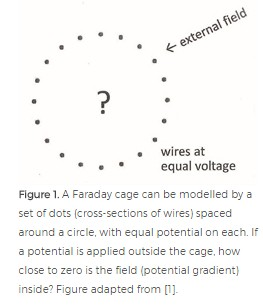# Surprises of the Faraday Cage

Lloyd N. Trefethen in Siam News:Nearly everyone has heard of the Faraday cage effect. So when I needed to learn about it, I assumed it would be a matter of looking in some standard physics books, maybe the ones I’d studied as an undergraduate. This was the beginning of a journey of surprises.

The Faraday cage effect involves shielding of electrostatic and electromagnetic fields. A closed metal cavity makes a perfect shield, with zero fields inside, and that is in the textbooks. Faraday’s discovery of 1836 was that fields are nearly zero inside a wire mesh, too. You see this principle applied in your microwave oven, whose front door contains a metal screen with small holes. The screen keeps the microwaves in, while allowing light, with its much smaller wavelength, to pass through.

The essence of the matter can be captured by a two-dimensional model (see Figure 1), where the cage is approximated by a circle or a line of dots representing cross-sections of wires all at the same voltage (connected somewhere in the third dimension). To keep things simple, we focus on electrostatic fields – the Laplace equation.

Let me explain how I got interested in this problem. André Weideman and I were finishing a survey of the trapezoidal rule for periodic analytic functions, which we’d been working on for eight years . We knew the mathematics of that problem: if ff is analytic and periodic and you add up sample values at equispaced points, you get an exponentially accurate approximation to its integral. Intuitively, sinusoidal oscillation in one direction corresponds to exponential decay in the direction at right angles in the complex plane. A contour integral estimate of Fourier coefficients exploits this decay to prove exponential accuracy.

To enrich our survey, I thought we should comment on the analogy between this mathematics of the trapezoidal rule and that of the Faraday cage. It seemed obvious the two must be related – it would just be a matter of sorting out the details.

More here.